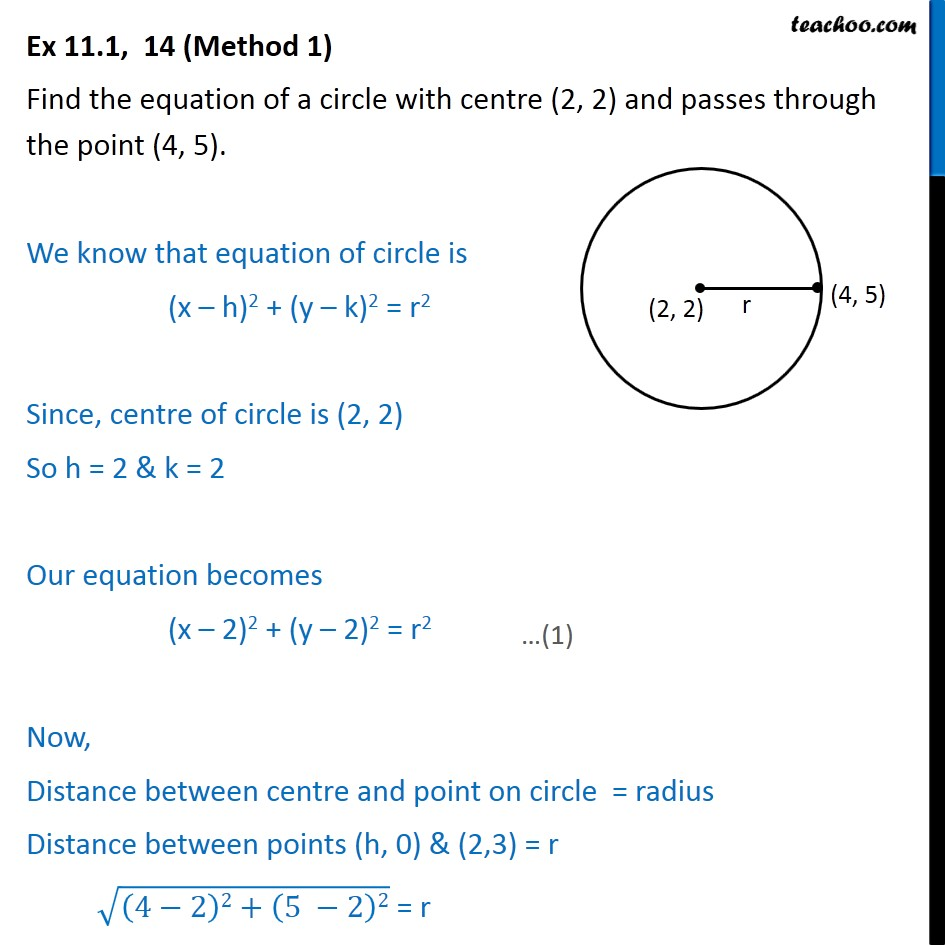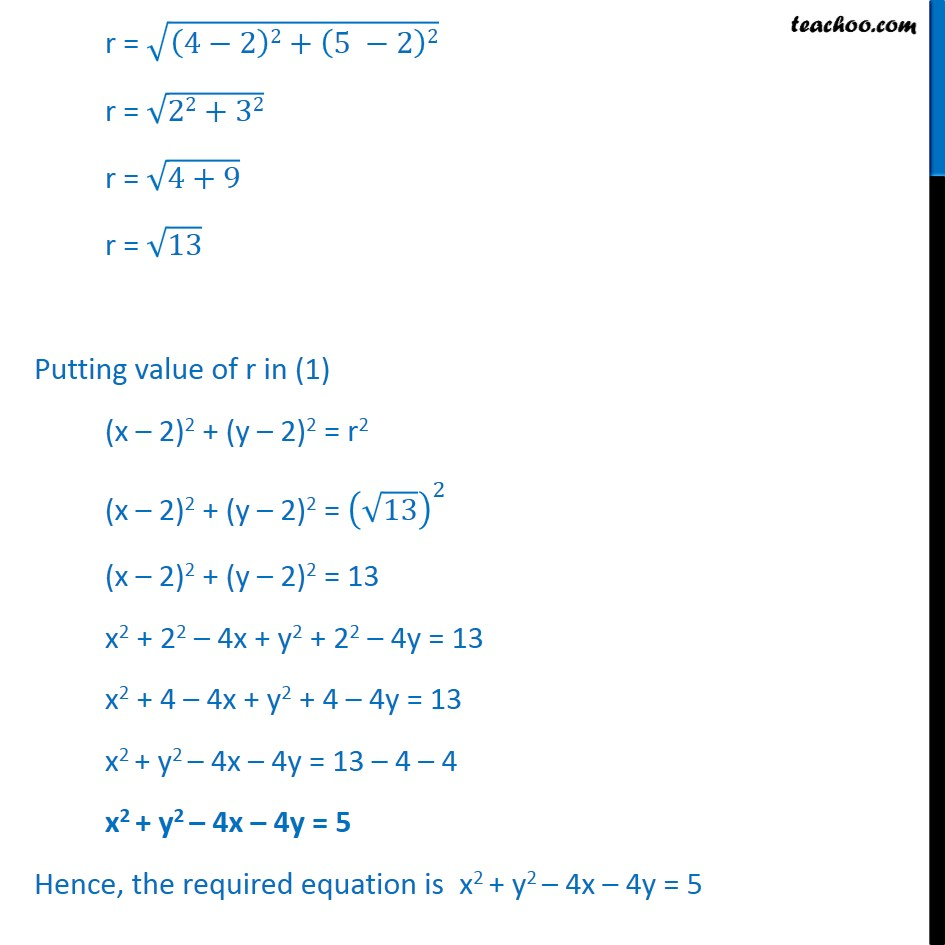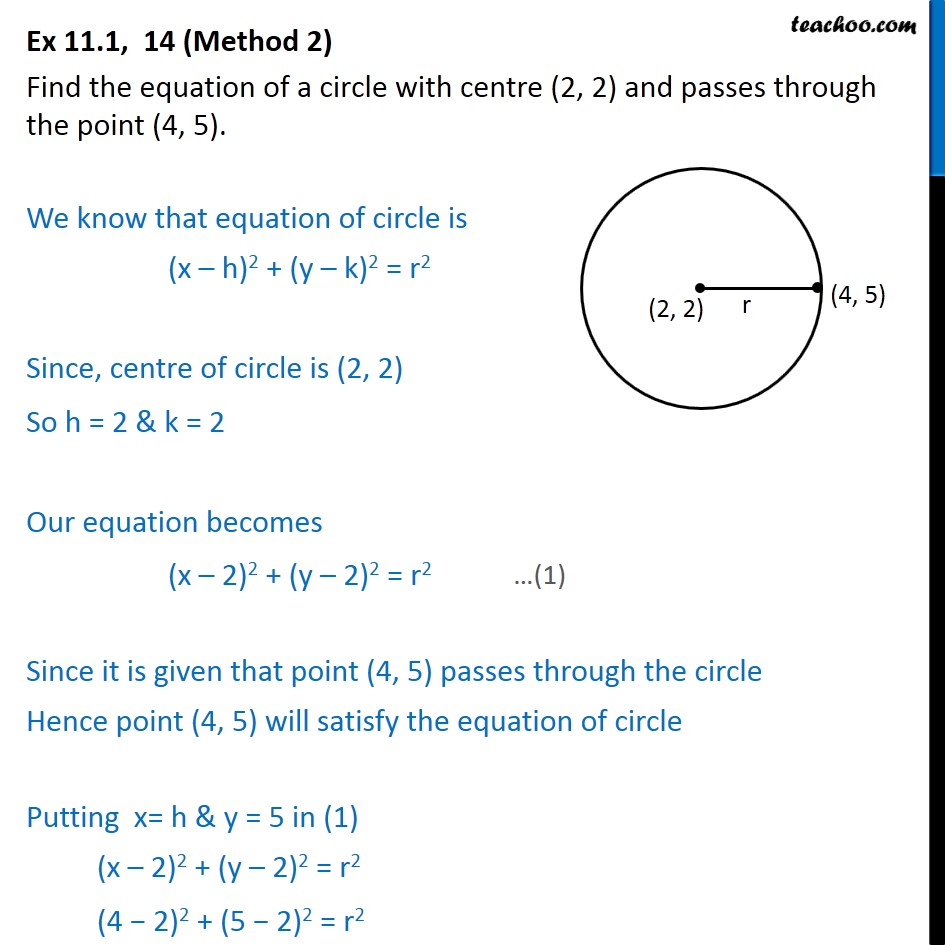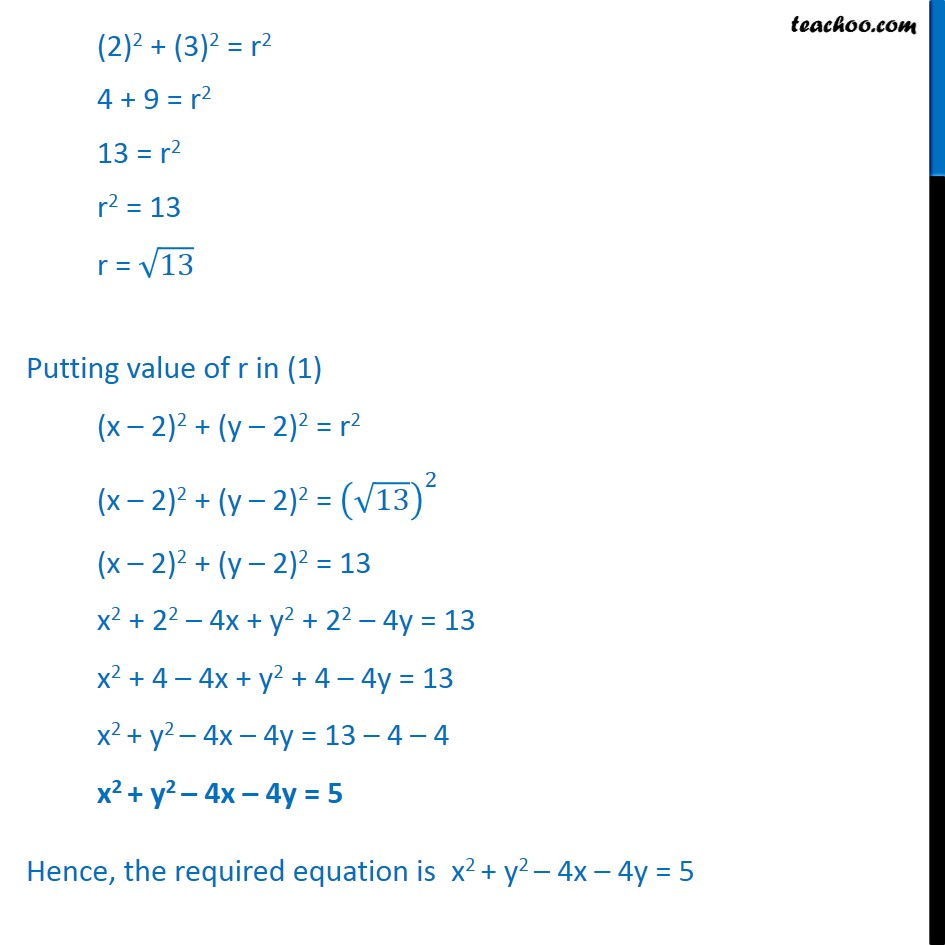Ex 10.1

Chapter 10 Class 11 Conic Sections
Serial order wiseLearn in your speed, with individual attention - Teachoo Maths 1-on-1 Class

### Transcript

Ex 10.1, 14 (Method 1) Find the equation of a circle with centre (2, 2) and passes through the point (4, 5). We know that equation of circle is (x – h)2 + (y – k)2 = r2 Since, centre of circle is (2, 2) So h = 2 & k = 2 Our equation becomes (x – 2)2 + (y – 2)2 = r2 Now, Distance between centre and point on circle = radius Distance between points (h, 0) & (2,3) = r ﷐﷮﷐4−2﷯2+﷐5 −2﷯2﷯ = r r = ﷐﷮﷐4−2﷯2+﷐5 −2﷯2﷯ r = ﷐﷮22+32﷯ r = ﷐﷮4+9﷯ r = ﷐﷮13﷯ Putting value of r in (1) (x – 2)2 + (y – 2)2 = r2 (x – 2)2 + (y – 2)2 = ﷐﷐﷐﷮13﷯﷯﷮2﷯ (x – 2)2 + (y – 2)2 = 13 x2 + 22 – 4x + y2 + 22 – 4y = 13 x2 + 4 – 4x + y2 + 4 – 4y = 13 x2 + y2 – 4x – 4y = 13 – 4 – 4 x2 + y2 – 4x – 4y = 5 Hence, the required equation is x2 + y2 – 4x – 4y = 5 Ex 10.1, 14 (Method 2) Find the equation of a circle with centre (2, 2) and passes through the point (4, 5). We know that equation of circle is (x – h)2 + (y – k)2 = r2 Since, centre of circle is (2, 2) So h = 2 & k = 2 Our equation becomes (x – 2)2 + (y – 2)2 = r2 Since it is given that point (4, 5) passes through the circle Hence point (4, 5) will satisfy the equation of circle Putting x= h & y = 5 in (1) (x – 2)2 + (y – 2)2 = r2 (4 − 2)2 + (5 − 2)2 = r2 (2)2 + (3)2 = r2 4 + 9 = r2 13 = r2 r2 = 13 r = ﷐﷮13﷯ Putting value of r in (1) (x – 2)2 + (y – 2)2 = r2 (x – 2)2 + (y – 2)2 = ﷐﷐﷐﷮13﷯﷯﷮2﷯ (x – 2)2 + (y – 2)2 = 13 x2 + 22 – 4x + y2 + 22 – 4y = 13 x2 + 4 – 4x + y2 + 4 – 4y = 13 x2 + y2 – 4x – 4y = 13 – 4 – 4 x2 + y2 – 4x – 4y = 5 Hence, the required equation is x2 + y2 – 4x – 4y = 5